• 今天小编就为大家分享一篇Python matplotlib 画图窗口显示到gui或者控制台的实例，具有很好的参考价值，希望对大家有所帮助。一起跟随小编过来看看吧
• 主要介绍了Python matplotlib画图时图例说明(legend)放到图像外侧详解，具有很好的参考价值，希望对大家有所帮助。一起跟随小编过来看看吧
• 主要介绍了解决Linux系统中python matplotlib画图的中文显示问题,需要的朋友可以参考下
• 在写论文时，如果是菜鸟级别，可能不会花太多时间去学latex，直接用...原因二：用matplotlib产生的图片太大，如果在word中显示就需要缩小，导致图片模糊，可以通过https://tinypng.com/ 该网站进行图片缩小再放入word
• 主要介绍了python matplotlib画图实例代码分享，具有一定借鉴价值,需要的朋友可以参考下
• 本文实例讲述了Python matplotlib画图与中文设置操作。分享给大家供大家参考，具体如下： 采用matplotlib作图时默认设置下是无法显示中文的，例如编写如下python脚本， #-*- coding: utf-8 -*- from pylab import *...
• import matplotlib.pyplot as plt # 代码中的“...”代表省略的其他参数 ax = plt.subplot(111) # 设置刻度字体大小 plt.xticks(fontsize=20) plt.yticks(fontsize=20) # 设置坐标标签字体大小 ax.xlabel(..., ...
• 主要介绍了Python matplotlib画图实例之绘制拥有彩条的图表，具有一定借鉴价值,需要的朋友可以参考下
• PythonMatplotlib画图基础介绍

千次阅读 2020-02-08 16:46:19
MatplotlibPython 中最常用的一个绘图库，主要用于绘制各种图形，包括散点图、柱状图、3D图、等高线图等等。在做研究过程中肯定会经常用，本文做一个简单的入门介绍，也给出极佳的参考手册，以备查用。内容主要...

Matplotlib 是 Python 中最常用的一个绘图库，主要用于绘制各种图形，包括散点图、柱状图、3D图、等高线图等等。在做研究过程中肯定会经常用，本文做一个简单的入门介绍，也给出极佳的参考手册，以备查用。内容主要参考了的内容，我自己用jupyter notebook实现了一遍，我会给出所有源码的文件。

重点推荐：https://matplotlib.org/gallery.html
该网页中收录了大量Matplotlib图形实例源码，基本上按需找到适当修改一下就行了，很棒！

除了Matplotlib之外再推荐一个高级统计画图工具：seaborn: statistical data visualization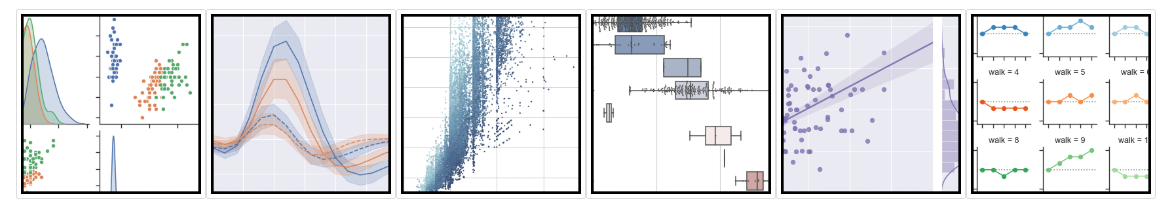Matplotlib第一个简单的图形

%matplotlib inline
import numpy as np
import matplotlib.pyplot as plt

plt.figure(0)
X = np.random.normal(size=(12, 2))
#plt.scatter(X[:, 0], X[:, 1])
plt.plot(X[:,0],X[:,1],'mo') #m表示紫色，o表示小圆点，更多颜色见文末
plt.show()坐标轴控制，标题

# create a new figure
plt.figure(1)
x = np.linspace(0, 2 * np.pi, 50)
y = np.sin(x)
plt.plot(x, y,label="sin(x)")
plt.plot(x, y * 2,label="2sin(x)")
plt.title("sin(x) & 2sin(x)") # 图像上方的标题
plt.xlim((0, np.pi*2)) # 横坐标的数值范围
plt.ylim((-3, 3)) # 纵坐标的数值范围
plt.xticks(np.linspace(0,np.pi*2,5)) # 认为指定在坐标轴上显示什么数字，我用linspace来画出5个值
#plt.xticks((0, np.pi * 0.5, np.pi, np.pi * 1.5, np.pi * 2))
plt.xlabel('XXX')  # 标记横轴坐标名称
plt.ylabel('YYY')  # 标记纵轴坐标名称
plt.legend(loc='upper right') # 标记标签，loc属性表示标签的位置，不写loc的话就是默认best，其他见下文
plt.show()legend loc参数选项：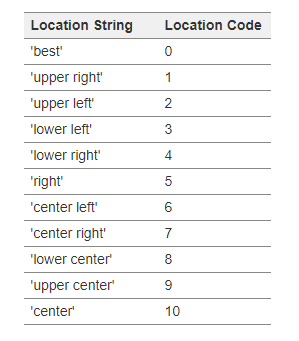颜色 表示方式
蓝色 b
绿色 g
红色 r
青色 c
品红 m
黄色 y
黑色 k
白色 w

点的类型 表示方式
点 .
像素 ,
圆 o
方形 s
三角形 ^

线的类型 表示方式
直线 -
虚线 –
点线 :
点划线 -

打上文字标注，公式

有时候我们需要对特定的点进行标注，我们可以使用 plt.annotate 函数来实现。这里我们要标注的点是(x0, y0) = (π, 0)。我们也可以使用 plt.text 函数来添加注释。

plt.plot(x, y)

x0 = np.pi
y0 = 0

# 画出标注点
plt.scatter(x0, y0, s=50)

plt.annotate('sin($\pi$)=%s' % y0, xy=(np.pi, 0), xycoords='data', xytext=(+30, -30),
textcoords='offset points', fontsize=16,

plt.text(0.5, -0.25, "$TEXTsin(\pi)=0$", fontdict={'size': 16, 'color': 'r'})

plt.show()

代码中的$\pi$是公式，参考latex公式去写就行了，很方便对于 annotate 函数的参数，做一个简单解释：
‘sin(np.pi)=%s’ % y0 代表标注的内容，可以通过字符串 %s 将 y0 的值传入字符串；
参数xycoords='data’是说基于数据的值来选位置;
xytext=(+30, -30) 和textcoords='offset points’表示对于标注位置的描述 和 xy 偏差值，即标注位置是 xy 位置向右移动 30，向下移动30；
arrowprops 是对图中箭头类型和箭头弧度的设置，需要用 dict 形式传入。

一个图中画多张子图

有时候我们需要将多张子图展示在一起，可以使用 subplot() 实现。即在调用 plot() 函数之前需要先调用 subplot() 函数。该函数的第一个参数代表子图的总行数，第二个参数代表子图的总列数，第三个参数代表活跃区域。

plt.figure(2)
ax1 = plt.subplot(2, 2, 1) # （行，列，活跃区）
plt.plot(x, np.sin(x), 'r+')

ax2 = plt.subplot(2, 2, 2) # 独立的y轴
plt.plot(x, 2 * np.sin(x), 'go')

ax3 = plt.subplot(2, 2, 3)
plt.plot(x, np.cos(x), '-b')

ax4 = plt.subplot(2, 2, 4, sharey=ax3) # 与 ax3 共享y轴
plt.plot(x, 2 * np.cos(x), 'y.')

plt.show()上面的 subplot(2, 2, x) 表示将图像窗口分为 2 行 2 列。x 表示当前子图所在的活跃区。

大小不一的多张子图

可以看到，上面的每个子图的大小都是一样的。有时候我们需要不同大小的子图。比如将上面第一张子图完全放置在第一行，其他的子图都放在第二行。

plt.figure(3)
ax1 = plt.subplot(2, 1, 1) # （行，列，活跃区）
plt.plot(x, np.sin(x), '-r+')

ax2 = plt.subplot(2, 3, 4)
plt.plot(x, 2 * np.sin(x), 'g')

ax3 = plt.subplot(2, 3, 5, sharey=ax2)
plt.plot(x, np.cos(x), 'b')

ax4 = plt.subplot(2, 3, 6, sharey=ax2)
plt.plot(x, 2 * np.cos(x), 'y')

plt.show()散点图

画一个炫炫的散点图：

k = 500
x = np.random.rand(k)
y = np.random.rand(k)
size = np.random.rand(k) * 50 # 生成每个点的大小
colour = np.arctan2(y, x) # 生成每个点的颜色大小
plt.scatter(x, y, s=size, c=colour)
plt.colorbar() # 添加颜色栏

plt.show()柱状图

常用的柱状图：

k = 10
x = np.arange(k)
y = np.random.rand(k)
plt.bar(x, y) # 画出 x 和 y 的柱状图

# 增加数值
for x, y in zip(x, y):
plt.text(x, y , '%.2f' % y, ha='center', va='bottom')

plt.show()plt.rcParams参数设置

plt（matplotlib.pyplot）使用rc配置文件来自定义图形的各种默认属性，称之为rc配置或rc参数。
通过rc参数可以修改默认的属性，包括窗体大小、每英寸的点数、线条宽度、颜色、样式、坐标轴、坐标和网络属性、文本、字体等。rc参数存储在字典变量中，通过字典的方式进行访问。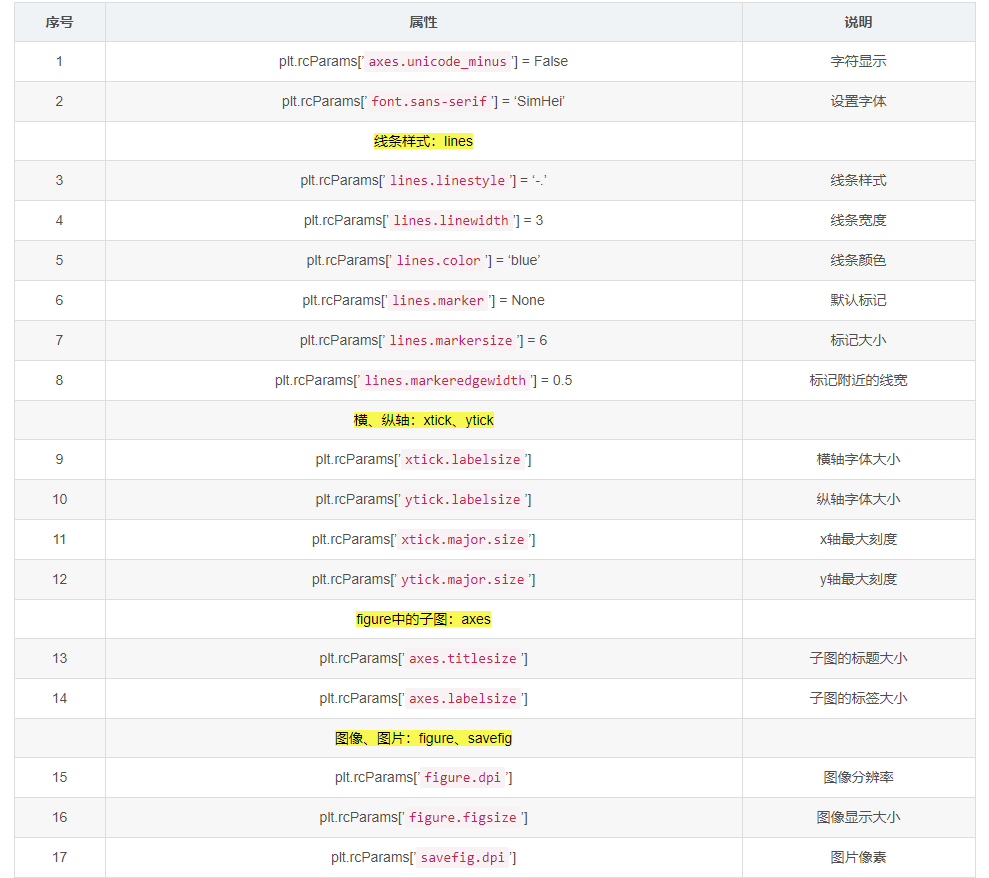详细颜色字母对照表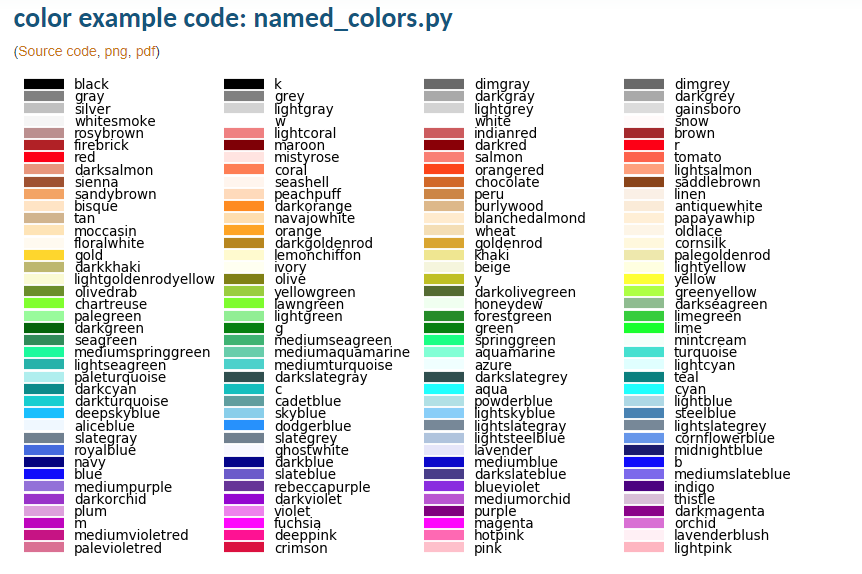参考资料

 https://www.jianshu.com/p/c41ac57cea33
 https://blog.csdn.net/weixin_39010770/article/details/88200298
 Matplotlib可视化及应用
 https://matplotlib.org/gallery.html

展开全文python 数据可视化 matplotlib
• python中使用matplotlib显示中文时,会遇到一些问题, 解决方案如下: import matplotlib.pyplot as plt # 支持中文 plt.rcParams['font.sans-serif'] = ['SimHei'] # 用来正常显示中文标签 plt.rcParams['axes.unicode...

python中使用matplotlib显示中文时,会遇到一些问题, 解决方案如下:

import matplotlib.pyplot as plt
# 支持中文
plt.rcParams['font.sans-serif'] = ['SimHei']  # 用来正常显示中文标签
plt.rcParams['axes.unicode_minus'] = False  # 用来正常显示负号

完整的例子

import matplotlib.pyplot as plt

# 支持中文
plt.rcParams['font.sans-serif'] = ['SimHei']  # 用来正常显示中文标签
plt.rcParams['axes.unicode_minus'] = False  # 用来正常显示负号

n_samples = 1500
random_state = 170
x, y = make_blobs(n_samples=n_samples, random_state=random_state)
# x, y = load_iris(True) # 莺尾花
print(x.shape, y.shape)
plt.scatter(x[:, 0], x[:, 1], c=y)
plt.title(u"原始数据分布")
plt.show()

Ref:

1. matplotlib完美中文显示
展开全文python 机器学习 数据分析 可视化 html
•技术
• 下面小编就为大家带来一篇基于Linux系统中python matplotlib画图的中文显示问题的解决方法。小编觉得挺不错的，现在就分享给大家，也给大家做个参考。一起跟随小编过来看看吧
• 使使用用python matplotlib 画画图图导导入入到到word 中中如如何何保保证证分分辨辨率率 这篇文章主要介绍了使用python matplotlib 画图导入到word中如何保证分辨率的例子具有很好的参考价值希 对大家有所帮助一起...
• import matplotlib.pyplot as plt ax = [] # 定义一个 x 轴的空列表用来接收动态的数据 ay = [] # 定义一个 y 轴的空列表用来接收动态的数据 plt.ion() # 开启一个画图的窗口 for i in range(100): # 遍历0-99的值 ...
• plt.plot(x,y) plt.title('figure') plt.xlabel('x') plt.ylabel('y')
plt.plot(x,y)
plt.title('figure')
plt.xlabel('x')
plt.ylabel('y')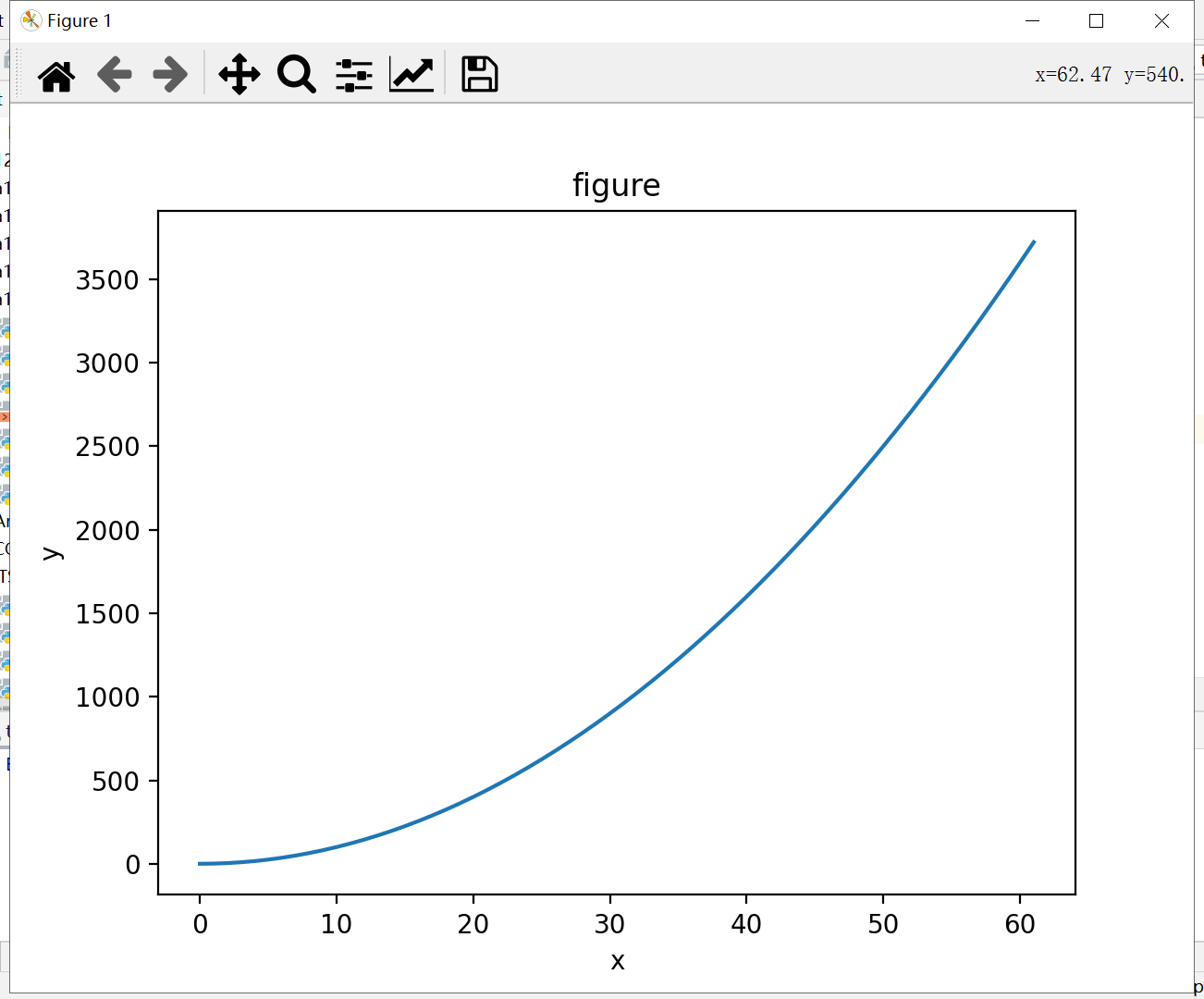展开全文python
• pythonmatplotlib画图

千次阅读 2021-12-13 11:19:15
引入matpltlib库 使用matpltlib库画图时...from matplotlib import pyplot as plt #生成数据 #横坐标数据从2017到2022，第三个参数可控制步长，可写可不写 x = range(2017, 2022) #y对应纵坐标的值 y1 = [49, 48, 45,

引入matpltlib库

matplotlib是一种优秀的python数据可视化第三方库
使用matpltlib库画图时，先将它引入，加载里面的pyplot，并命名为plt，然后使用plot函数画图

import matplotlib.pyplot as plt #plt是引入模块的别名

pyplot基础图标函数总结

函数作用
plt.plot(x,y,format)绘制坐标图
plt.plot(data,notch,position)绘制箱型图
plt.bar(left,height,width,bottom)绘制条形图
plt.barh(width,bottom,left,height)绘制横向条形图
plt.polar(theta,r)绘制极坐标图
plt.pie(data,explode)绘制饼图
plt.hist(x,bins,normed)绘制直方图
plt.scatter(x,y)绘制散点图

还有很多哈哈哈，不再注意列举

plot函数画图语法规则

plot函数参数：plot([x],y,[format],**kwargs)

参数用途
xx轴的数据，列表或者数组
yy轴数据，列表或数组
format控制曲线的格式字符串
**kwargs第二组或更多（x,y,string）

各类语法太多啦，偷几张MOOC的图放上来~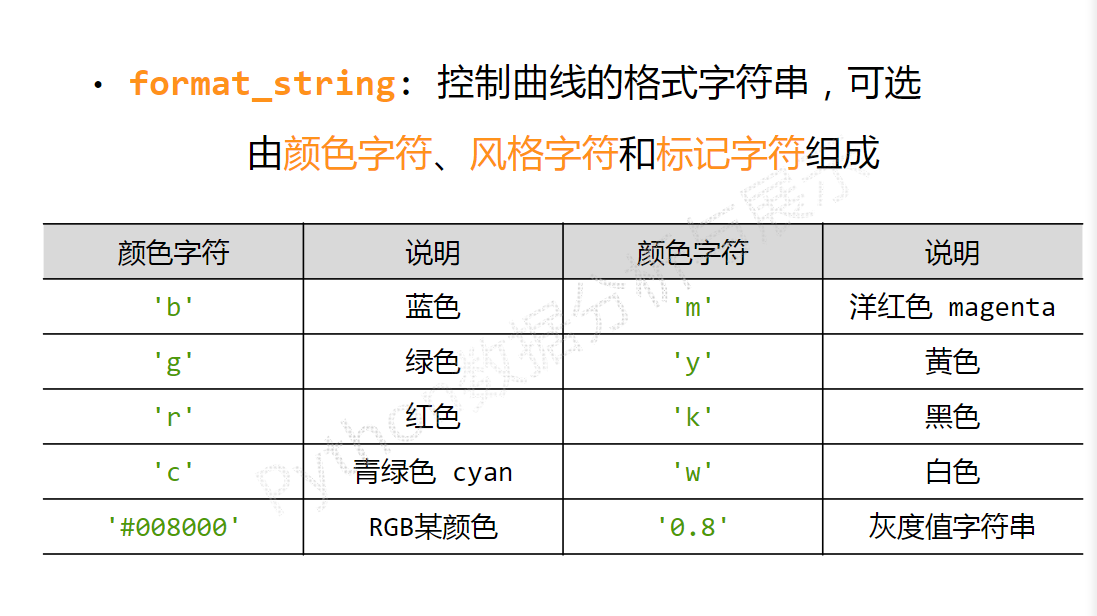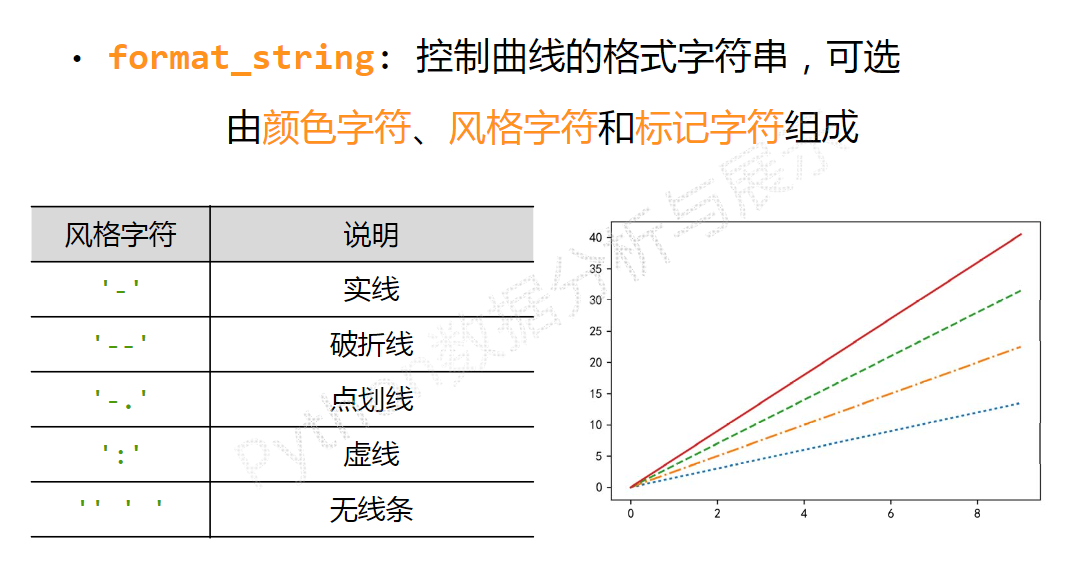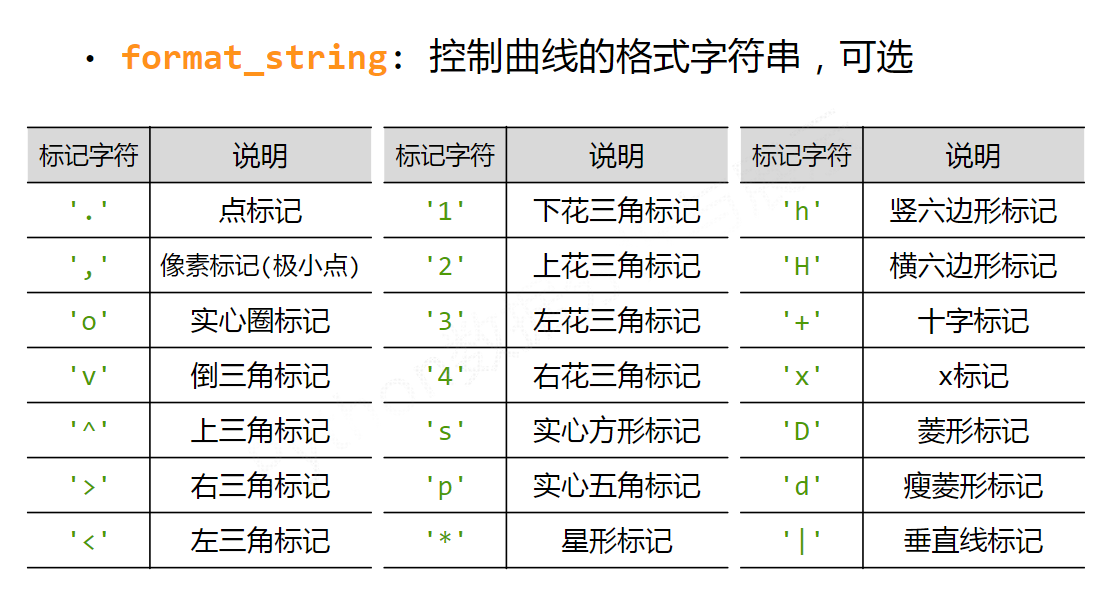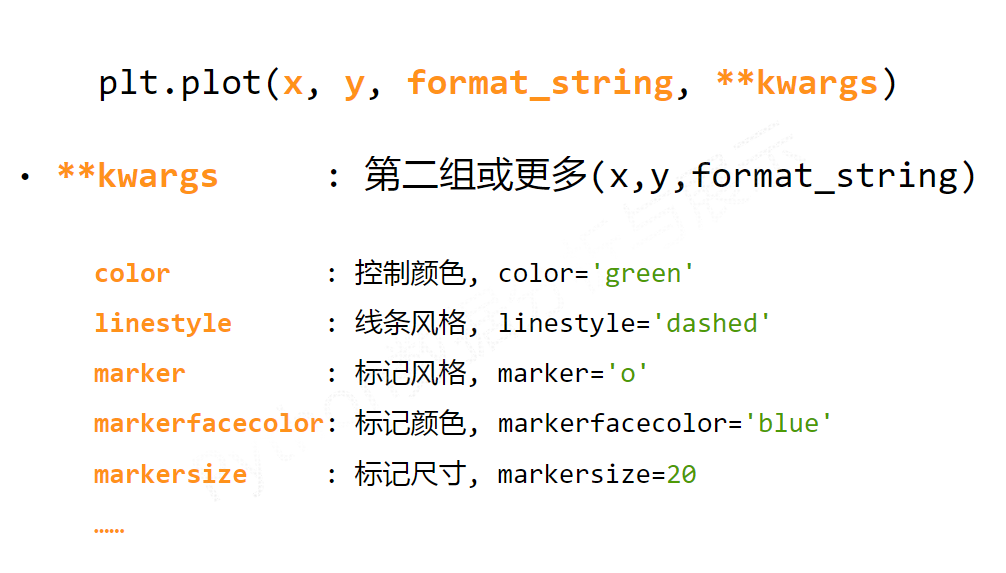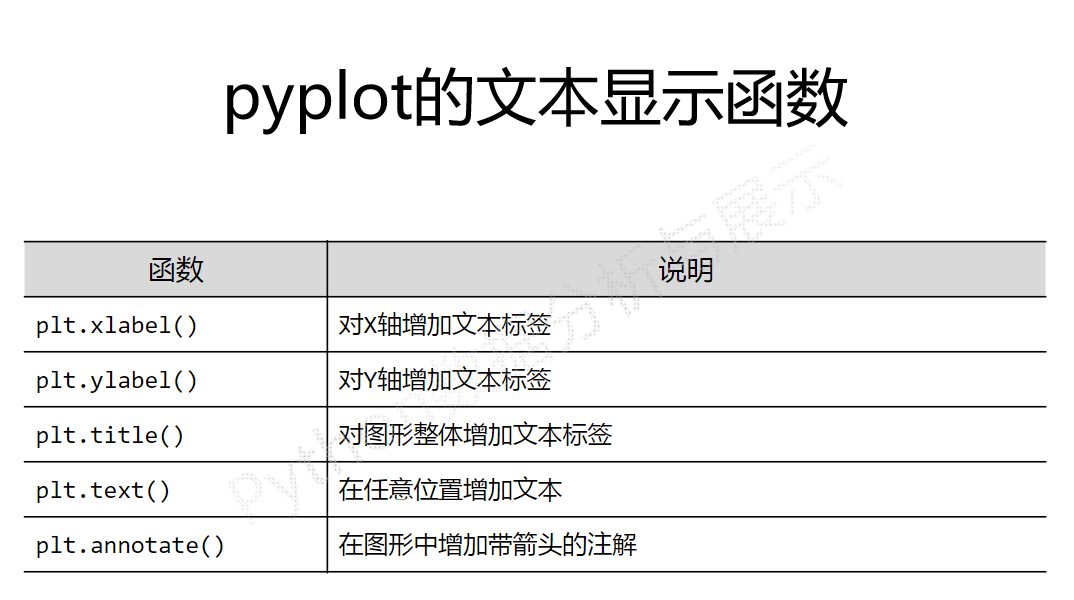折线图

from matplotlib import pyplot as plt

#生成数据
#横坐标数据从2017到2022，第三个参数可控制步长，可写可不写
x = range(2017, 2022)
#y对应纵坐标的值
y1 = [49, 48, 45, 52, 50]
y2 = [60, 62, 61, 65, 63]
#生成图形
plt.title("LMY and her mother's weight")
plt.xlabel('year')
plt.ylabel('kg')
plt.plot(x, y1, color='green', label='LMY')
plt.plot(x, y2, color='purple', label='mother')
plt.grid(alpha=0.5)
plt.legend(loc='upper right')
#显示图形
plt.show()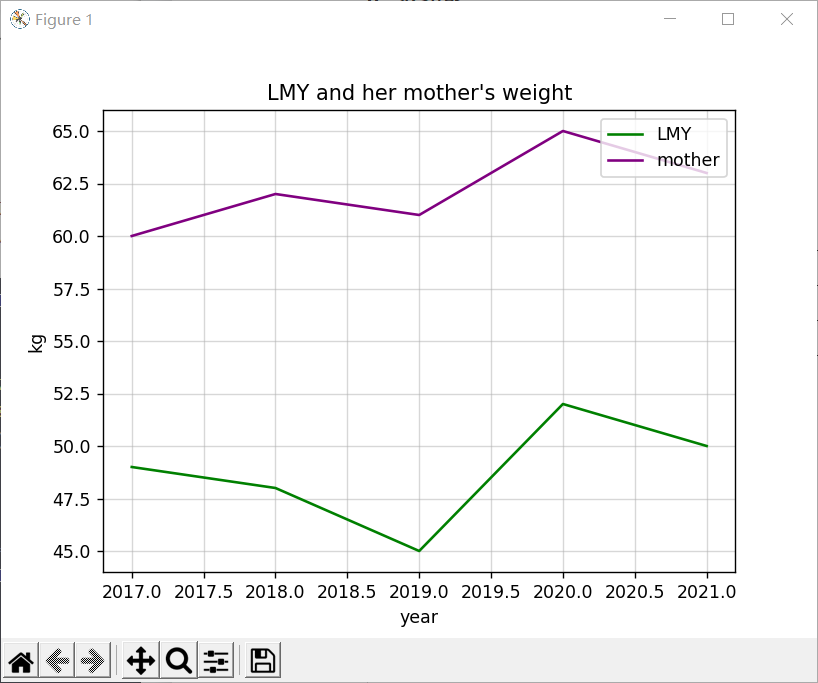散点图

from matplotlib import pyplot as plt
import numpy as np

# 生成数据
# 横坐标数据从20172022，第三个参数可控制步长，可写可不写
x = range(2017, 2022)
# y对应纵坐标的值
y1 = [49, 48, 45, 52, 50]
y2 = [60, 62, 61, 65, 63]
# 生成图形
plt.title("LMY and her mother's weight")
plt.xlabel('year')
plt.ylabel('kg')
# 点的大小
area = np.pi*4**2
plt.scatter(x, y1, s=area, c='yellow', alpha=1)
plt.scatter(x, y2, s=area, c='blue', alpha=1)
plt.legend()
plt.yticks(())
plt.show()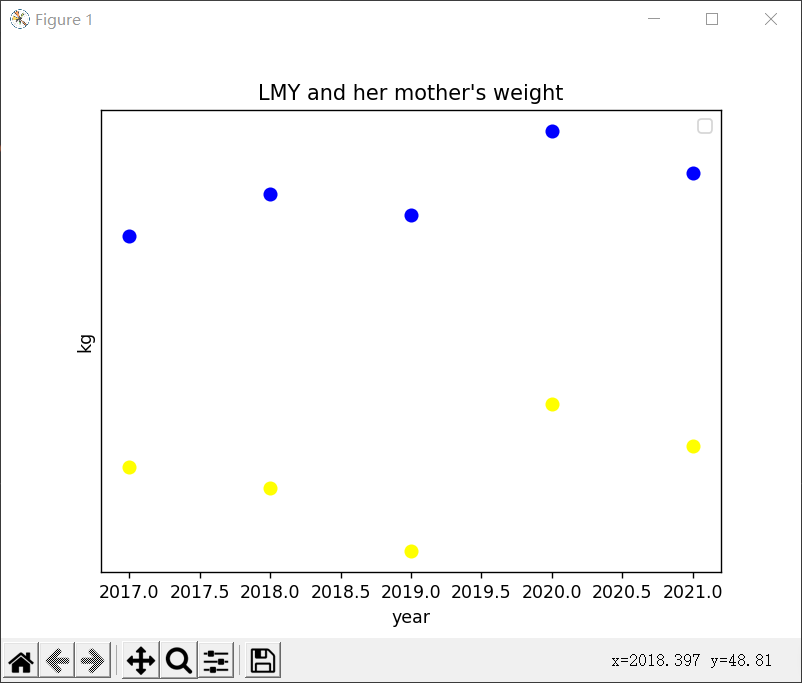直方图

from matplotlib import pyplot as plt
import numpy as np

# 生成数据
# 横坐标数据从20172022，第三个参数可控制步长，可写可不写
x = [2017, 2018, 2019, 2020, 2021]
# y对应纵坐标的值
y1 = [49, 48, 45, 52, 50]
y2 = [60, 62, 61, 65, 63]
# 生成图形
plt.title("LMY and her mother's weight")
plt.ylabel('frequency')
plt.xlabel('kg')
# 点的大小
plt.hist(y1, bottom=None, color='purple')
plt.hist(y2, bottom=None, color='pink')
plt.show()

# n, bins, patches = plt.hist(arr, bins=50, normed=1, facecolor='green', alpha=0.75)
'''
arr:需要计算直方图的一维数组
bins:直方图的柱数，可选项，默认为10
normed:是否将得到的直方图向量归一化，默认为0
facecolor:直方图颜色
alpha:透明度
'''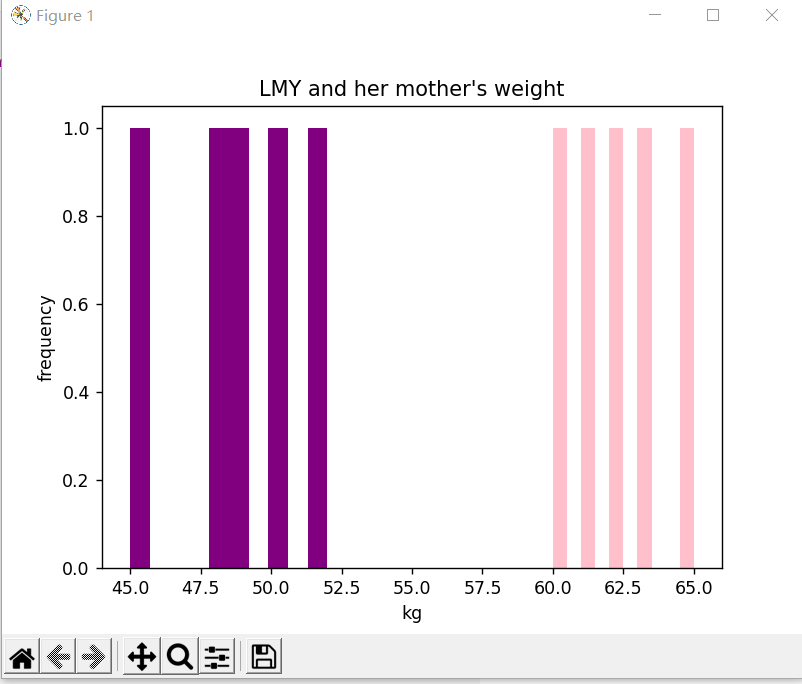条形图

纵向

from matplotlib import pyplot as plt
import numpy as np

arr = np.arange(2017, 2022)
x = [49, 48, 45, 52, 50]  # x轴
y = [2017, 2018, 2019, 2020, 2021]
rect = plt.bar(arr, x, width=0.5)
plt.title('LMY')
plt.xlabel('weight')
plt.ylabel('year')
plt.legend()

plt.show()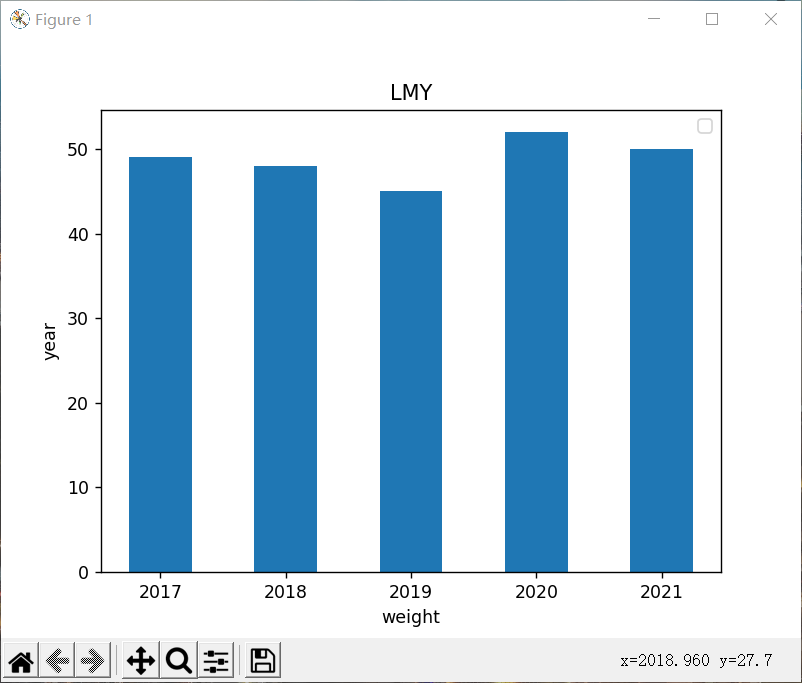横向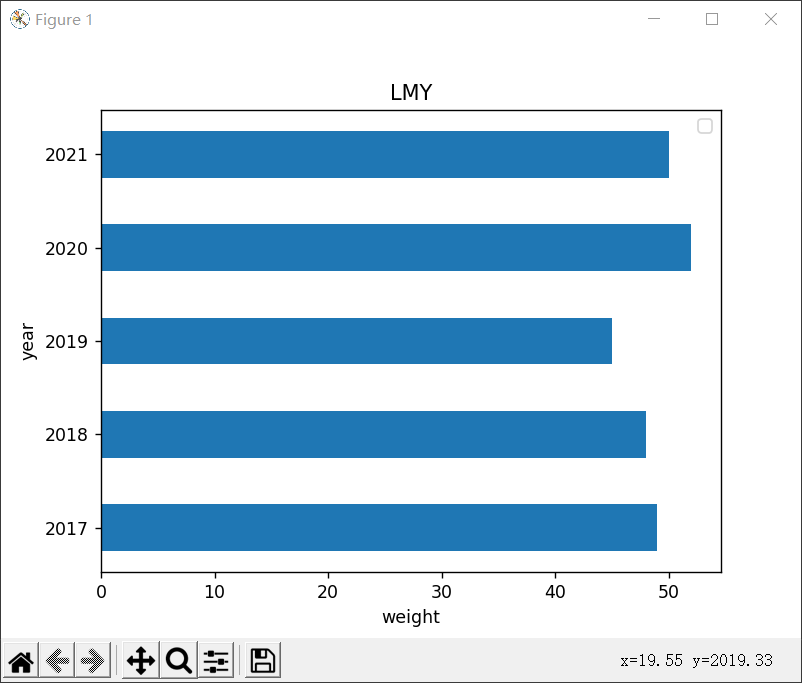多条

from matplotlib import pyplot as plt
import numpy as np

arr = np.arange(2017, 2022)
x1 = [49, 48, 45, 52, 50]  # x轴
x2 = [60, 62, 61, 65, 63]
y = [2017, 2018, 2019, 2020, 2021]
rects1 = plt.bar(arr, x1, 0.5, color='purple', label='LMY')
rects2 = plt.bar(arr, x2, 0.5, color='yellow', label='Mother', alpha=0.3)
plt.title("LMY and her mother's weight")
plt.xlabel('weight')
plt.ylabel('year')
plt.legend()

plt.show()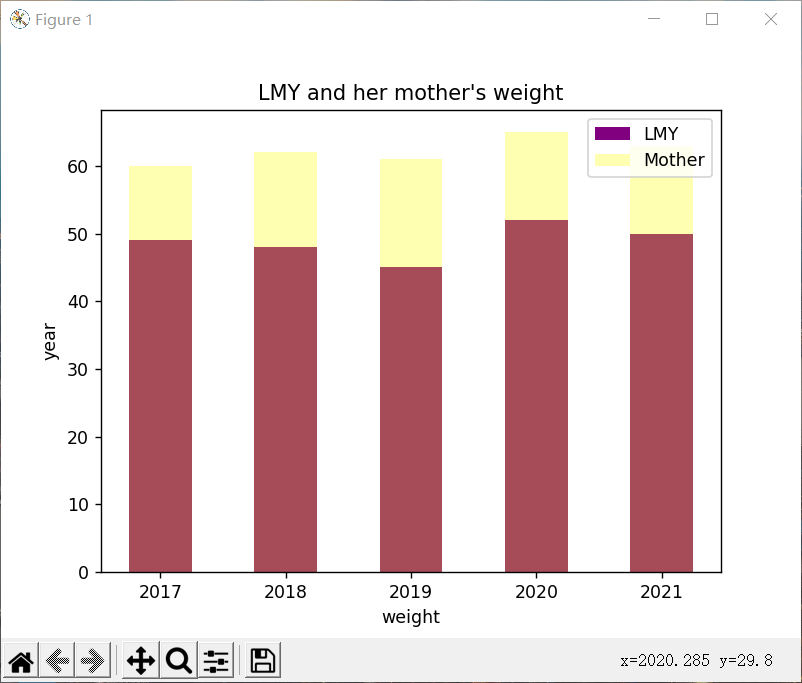饼图

from matplotlib import patches, pyplot as plt
import numpy as np

label_list = ['49', '48', '45', '52', '50']
size = [20, 20, 20, 20, 20]
# 各部分的颜色
color = ['red', 'pink', 'blue', 'green', 'purple']
explode = [0, 0, 0.15, 0, 0]

patches, l_text, p_text = plt.pie(size, explode=explode, colors=color, labels=label_list,
plt.axis('equal')
plt.title("LMY's weight")
plt.legend(loc='upper left')
plt.show()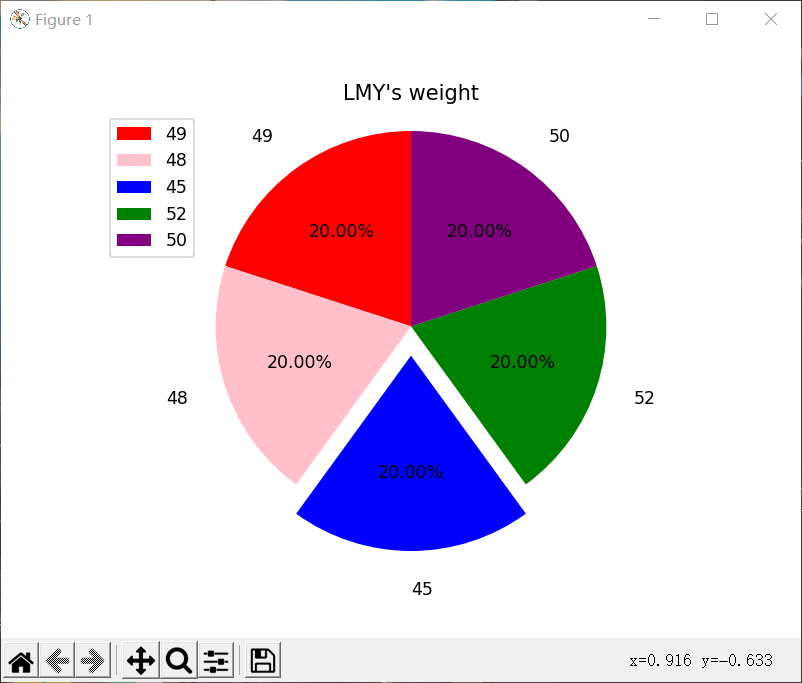展开全文python 机器学习 矩阵 matplotlib 可视化
• 用pythonmatplotlib画图，无法显示图像

万次阅读 多人点赞 2018-07-09 11:12:52
最近在看《机器学习实战》这本书，也就是《Machine Learning in Action》的中文版，看到第13章 利用PCA来简化数据，跑了一下书里的代码，死活就是...所以用matplotlib画图，一定记得最后加上这句话，否则不出图哈......

pythonmatplotlib画图python 订阅